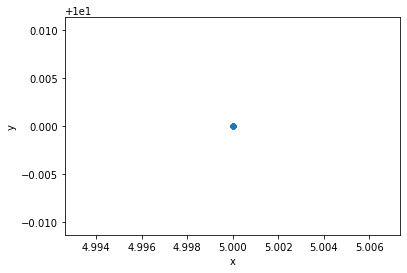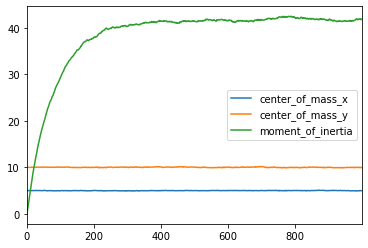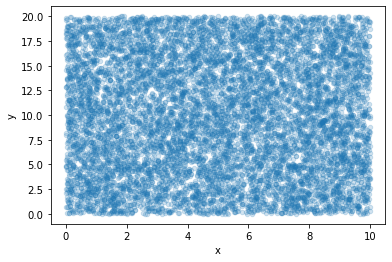# Distribute¶

Now that we have separated our problem, let's distribute the pieces.

We'll use Dask.distributed for distributed computing and actors. More on actors here.

We'll have four workers with 1 thread each that will host different parts of our physical model.

In :
from dask.distributed import Client

client

Out:

### Cluster

• Workers: 3
• Cores: 3
• Memory: 3.00 GB

## Space, particles, and random numbers¶

The following is largely identical to what we did in 02_separation-Copy1.ipynb.

In :
%matplotlib inline
import numpy as np
import pandas as pd


The spatial domain doesn't call anything. It's identical to the earlier implementation.

In :
class PeriodicSpace:
def __init__(self, length_x=10, length_y=20):
self.length_y = length_y
self.length_x = length_x

def get_sizes(self):
return self.length_x, self.length_y

def normalize_positions(self, x, y):
return np.mod(x, self.length_x), np.mod(y, self.length_y)


Each particle calls the spatial domain. In distributed mode, we'll have to make sure that we get the .result() from the Future objects returned when calling methods on actors.

In :
class Particles:
def __init__(
self,
rng=np.random.RandomState(),
space=PeriodicSpace(),
x=None, y=None,
step_length=0.5
):
self.rng = rng
self.space = space
self.x, self.y = x, y
self.step_length = step_length
self.steps_done = 0

def move(self):
self.x += self.step_length * self.rng.normal(size=self.x.shape).result()
self.y += self.step_length * self.rng.normal(size=self.y.shape).result()

self.x, self.y = self.space.normalize_positions(self.x, self.y).result()

self.steps_done += 1

def center_of_mass(self):
return self.x.mean(), self.y.mean()

def moment_of_inertia(self):
return self.x.var() + self.y.var()

def diagnostics(self):
com = self.center_of_mass()
mi = self.moment_of_inertia()
return pd.DataFrame(
{
"center_of_mass_x": com,
"center_of_mass_y": com,
"moment_of_inertia": mi
},
index=[self.steps_done, ],
)

def positions(self):
return pd.DataFrame(
{
"x": self.x,
"y": self.y
}
)


## Submit space, rng and group of particles to the cluster¶

In :
space = client.submit(
PeriodicSpace,
length_x=10,
length_y=20,
actor=True,
pure=False
).result()

In :
rng = client.submit(
np.random.RandomState, actor=True, pure=False
).result()

In :
length_x, length_y = space.get_sizes().result()
particles = client.submit(
Particles, space=space, rng=rng,
x=np.ones((10_000, )) * length_x / 2.0,
y=np.ones((10_000, )) * length_y / 2.0,
actor=True, pure=False
).result()


## Plot initial positions¶

In :
particles.positions().result().plot.scatter(x="x", y="y");## Run the main loop¶

In :
diags = particles.diagnostics().result()

for step in range(1, 1000):
particles.move().result()

diags = diags.append(
particles.diagnostics().result()
)


## Plot data¶

In :
diags.plot();In :
particles.positions().result().plot.scatter(x="x", y="y", alpha=0.2);